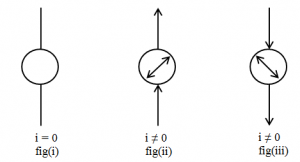# Orested Discovery

The Danish scientist Orested established relation between electric and magnetic field. On his experiment when he placed the current carrying conductor parallel to the compass needle it was interestingly found that there was deflection of magnetic needle. When the direction of current was reversed then the deflection of compass needle was in opposite direction.
From his experiment he concluded that there is relation between magnetic field and electric field.In fig(i) there is no deflection of compass needle due to the absence of current. In fig(ii) there is deflection of compass needle due to the current on the conductor. In fig(iii) the is deflection of compass needle to the opposite direction in reversing the direction of current.

Direction of magnetic field and current:
There are different rule to determine the direction of magnetic field and current.

Right hand thumb rule:
This rule is used to determine the direction of magnetic field due to the straight current carrying conductor. According to this rule, if thumb of right hand represent the direction of straight current carrying conductor then tips of the curve figures represent the direction of magnetic field.

Maxwell’s croke screw rule (Right hand screw rule):
This rule is also used to determine the direction of magnetic field due to current carrying conductor. According to this rule, when the screw is rotated in the right hand side the forward motion of the screw represent the direction of the current carrying conductor and the circular motion of the screw represent the direction of magnetic field.

Right hand fist rule:
This rule is used to determine the direction of magnatic field due to circular current carrying coil. According to this rule, if the tips of the curved fingures represent the direction of current on the circular coil then the thumb of the right hand represent the direction of magnatic field.

Fleming’s left hand rule:
This rule is used to determine the direction of magnatic field, motion of charged oarticle on magnatic field and force exerted on the magnatic field. According to this rule, if the thumb, fore and middle finger of the left hand are mutually perpendicular to eachother then the middle figure represent the direction of motion of charged particles on the magnatic field, fore finger represent the direction of magnatic field and thumb finger represents the direction of magnatic force.